Evaluation Hardware \$72.60## Design Resources

### Design & Integration Files

• Schematic
• Bill of Materials
• Gerber Files
• Assembly Drawing

### Evaluation Hardware

Part Numbers with "Z" indicate RoHS Compliance. Boards checked are needed to evaluate this circuit.

• EVAL-SDP-CB1Z (\$116.52) Eval Control Board

### Device Drivers

Software such as C code and/or FPGA code, used to communicate with component's digital interface.

## Features & Benefits

• 40GHz Power Meter
• 45dB Dynamic Range
• Low Power

## Circuit Function & Benefits

The circuit shown in Figure 1 is an accurate 40 GHz, microwave power meter with a 45 dB range that requires only two components. The RF detector has an innovative detector cell using Schottky diodes followed by an analog linearization circuit. A low power, 12-bit, 1 MSPS analog-to-digital converter (ADC) provides a digital output on a serial peripheral interface (SPI) port.

A simple calibration routine is run before measurement operation, at the particular RF frequency of interest. The user can then operate the system in measurement mode. When in measurement mode, the CN-0366 Evaluation Software displays the calibrated RF input power that is applied at the input of the detector in units of dBm.

The total power dissipation of this circuit is less than 9 mW on a single 5 V supply.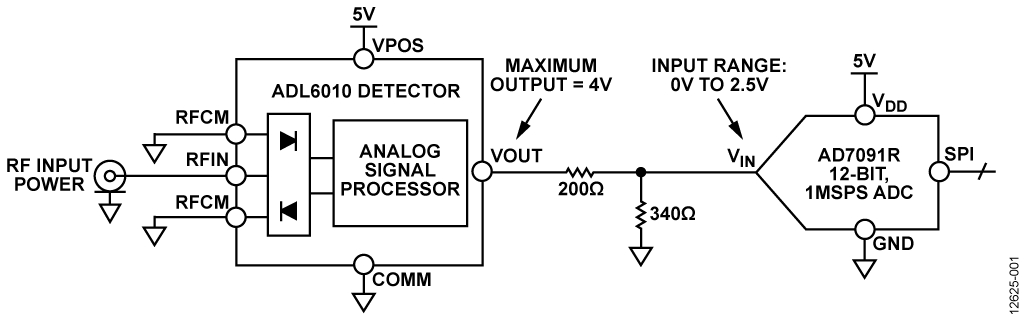## Circuit Description

The circuit shown in Figure 1 uses an ADL6010 RF and microwave power detector to convert an ac waveform to a scaled output voltage that corresponds to the amplitude of the input waveform. The output voltage is linear-in-voltage, having a slope with units of V/V rms. The ADL6010 can extract RF signal envelopes with bandwidths of up to 40 MHz. However, in most power meter applications, the output voltage is a settled dc value that represents the amplitude of the input waveform.

The AD7091R 12-bit, 1 MSPS ADC samples the detector output, and the data is processed through a data capture board and sent to a PC for further processing and analysis. The ADC has an internal 2.5 V reference voltage that can be used to set the full-scale voltage. The internal reference can be overridden if a larger full-scale voltage is needed.

The system must be calibrated because the output voltage is dependent on the frequency of the input waveform. A correction factor is also needed when measuring modulated signals. PC based software with a simple graphical user interface is provided to perform the computations (CN-0366 Evaluation Software).

### Power Detector

The ADL6010 is 45 dB envelope detector that operates from 500 MHz to 43.5 GHz. It has a linear in volts slope of approximately 5.9 V/V rms and an absolute detector input range from −30 dBm to +15 dBm or −43 dBV to +2 dBV in a 50 Ω system. The detector cell uses a proprietary eight Schottky diode array followed by a novel linearizer circuit that creates a linear voltmeter with an overall scaling factor (or transfer gain) of nominally ×5.9 relative to the rms voltage amplitude of the input. With an output averaging capacitor, the ADL6010 can detect a signal with a varying envelope, but a correction factor must be used to compensate for the change in output voltage for the same given input power. The output voltage is related to the rms input voltage bywhere:

VOUT is the voltage on the VOUT pin.
Slope is approximately 5.9 V/V rms at 10 GHz.
VRFIN is the rms input voltage.
Intercept is the y-axis value that the data crosses if extended.

Figure 2 shows a functional block diagram of the ADL6010.

Figure 3 shows the variation in the transfer function with frequency. There is approximately 300 mV of voltage deviation in the output between 1 GHz and 40 GHz. The temperature variation is less than ±0.5 dB over the entire frequency range. Figure 4 and Figure 5 show the temperature variation at 10 GHz and 40 GHz, respectively.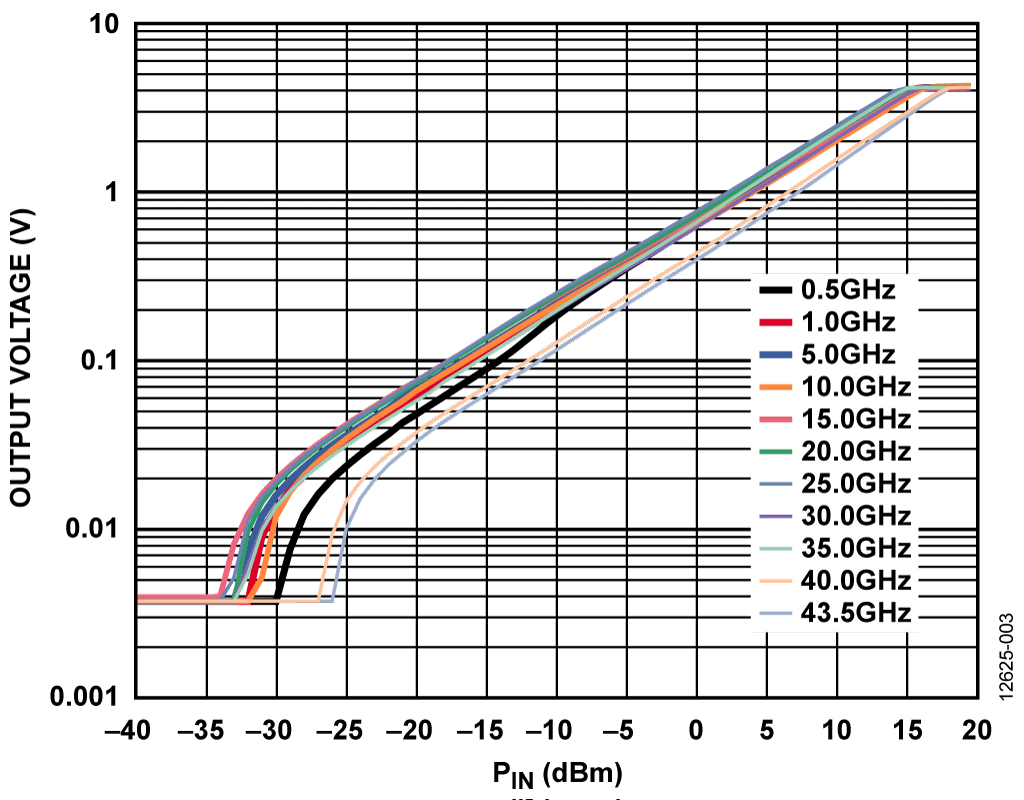Figure 3. Transfer Function at Frequencies from 500 MHz to 43.5 GHzFigure 4. Transfer Function and Error at 10 GHZ for Various TemperaturesFigure 5. Transfer Function and Error at 40 GHz for Various Temperatures

### Analog-to-Digital Converter

The AD7091R is a 12-bit, 1 MSPS ADC with an input voltage range between 0 V and VREF, where the reference voltage is either provided by the internal 2.5 V reference or by an external reference that overrides the internal reference. The external reference can be as high as 5 V. For a 2.5 V full-scale voltage (VREF = 2.5 V), the LSB size isThe output voltage of the ADL6010 is approximately 25 mV to 4 V; therefore, a 200 Ω/340 Ω resistor divider with an attenuation of approximately 1.6 reduces the amplitude of the signal so that it is always within the range of the AD7091R when using the internal 2.5 V reference.

### Data Analysis

The EVAL-SDP-CB1Z system demonstration platform (SDP) board is used in conjunction with software based on the AD7091R evaluation board control software to capture the data being sampled by the ADC. The software has a power meter readout and calibration option. The power meter display shows the power applied to the input of the ADL6010. To take an accurate power measurement with the ADL6010 and the AD7091R, apply two known input powers at different levels to the input of the ADL6010, then read the corresponding output ADC code. These four values make up two points on a plot and must be stored for later use in the calibration procedure. The two points are

• Point 1: (VLOW, CODELOW)
• Point 2: (VHIGH, CODEHIGH)

From these two points, a slope and an intercept can be found and used to calibrate the system at the particular frequency of operation.

Figure 6 shows the software power level display.

### System Transfer Function

The slope and intercept of the system from the input of the detector to the output of the ADC arewhere:

SlopeSYS is the system slope.
CODEHIGH, CODELOW are the high and low code outputs, respectively, from the ADC.
VHIGH, VLOW are the high and low RF voltages, respectively.
INTSYS is the system intercept.

The overall system transfer function is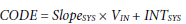where VIN is the rms voltage of the input RF signal.

Solve for VIN using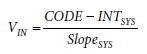Therefore, the power in dBm, PIN, can be expressed asFor a 50 Ω input impedance, this equation simplifies to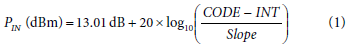### User Calibration Algorithm

The CN-0366 Evaluation Software performs a one-time calibration at the particular frequency of operation. Calibration is achieved via the Calibration tab, shown in the window of Figure 6. The calibration routine is as follows:

1. Set the RF power to high level (VHIGH).
2. Measure the code from ADC (CODEHIGH).
3. Set the RF power to low level (VLOW).
4. Measure the code from ADC (CODELOW).
5. Calculate the system slope (units of codes/V).
6. Calculate the system intercept (units of codes).
7. Store the slope and intercept as calibration coefficients.
8. Measure the ADC code with an arbitrary input RF power.
9. Calculate the input power using the code, slope, and intercept.

### Measurement Results for Complete Signal Chain Including ADC

Using the CN-0366 Evaluation Software, measurements were taken across several frequencies. Each frequency was calibrated before the measurement took place. The results are shown in Figure 7, Figure 8, and Figure 9. In Figure 7, note the dependency of the error on the calibration power levels. Choosing the proper calibration levels may require some trial and error.

Figure 7 through Figure 9 show the input power measured vs. the actual power applied to the input of the ADL6010 and the error between the two. Data was taken over temperature at 0°C, 25°C, and 70°C, and the results are shown in Figure 10, Figure 11, Figure 12, and Figure 13 for frequencies of 1 GHz, 10 GHz, 20 GHz, and 30 GHz. Note that some measurements were taken using an early version of the software that did not have an averaging feature, which is why there is a large ripple at the lower input power levels.Figure 7. Measured Power and Error vs. Applied Power for Various Three-Point Calibrations at 1 GHzFigure 9. Measured Power Error vs. Applied Power at Various FrequenciesFigure 10. Measured Power and Error vs. Applied Power for Various Temperatures at 1 GHz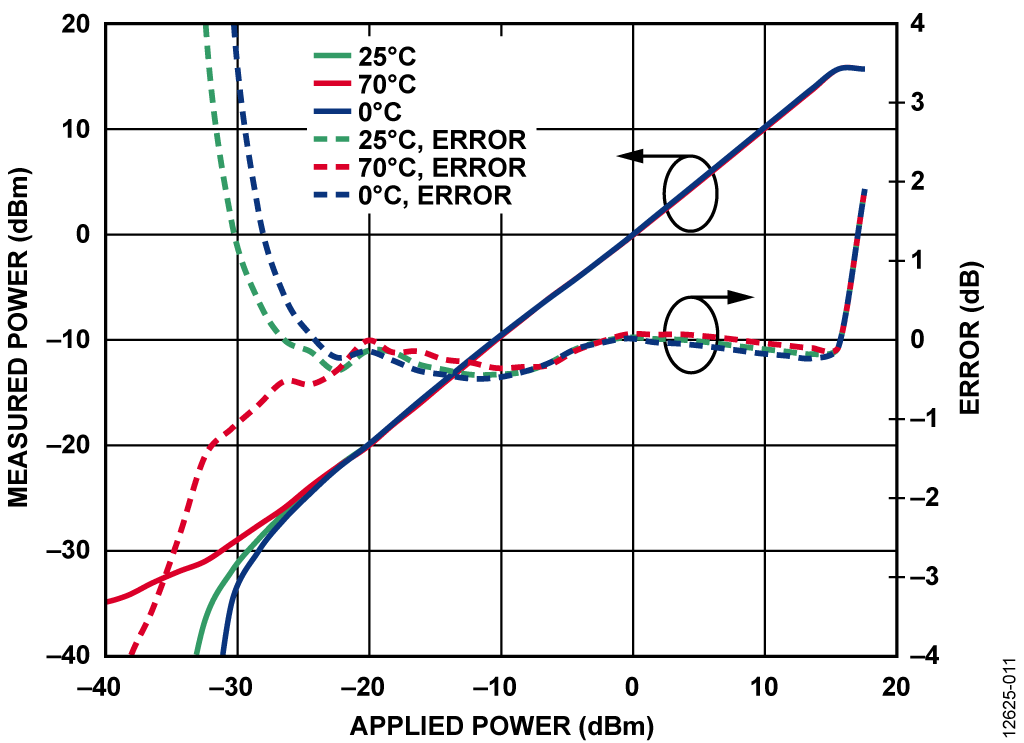Figure 11. Measured Power and Error vs. Applied Power for Various Temperatures at 10 GHz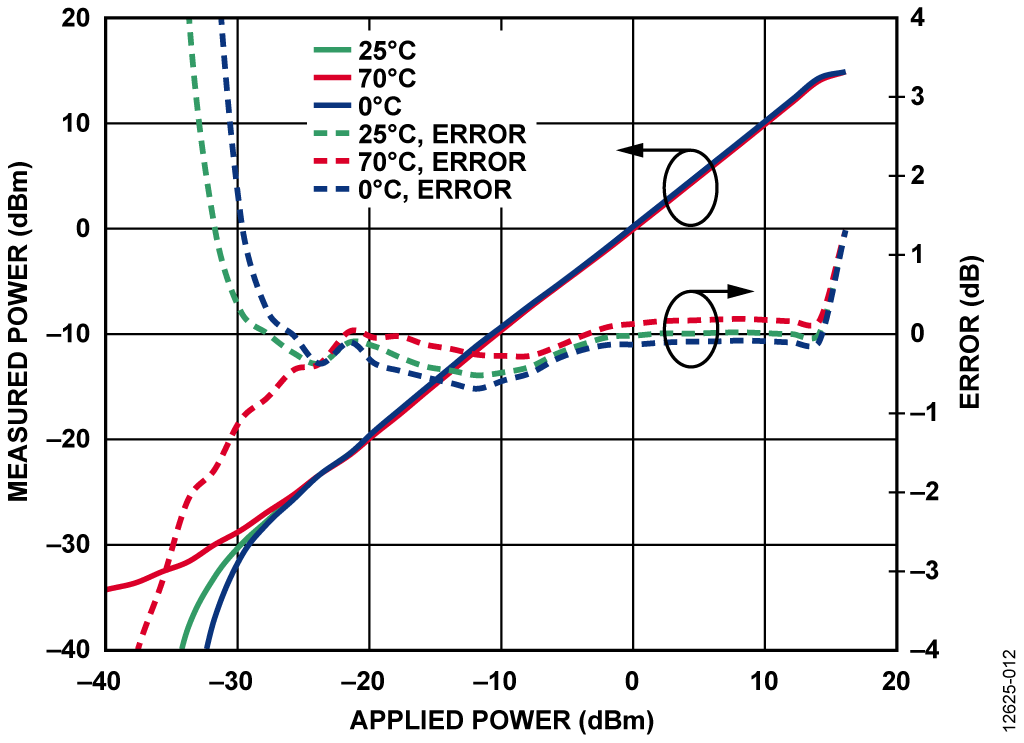Figure 12: Measured Power and Error vs. Applied Power for Various Temperatures at 20 GHz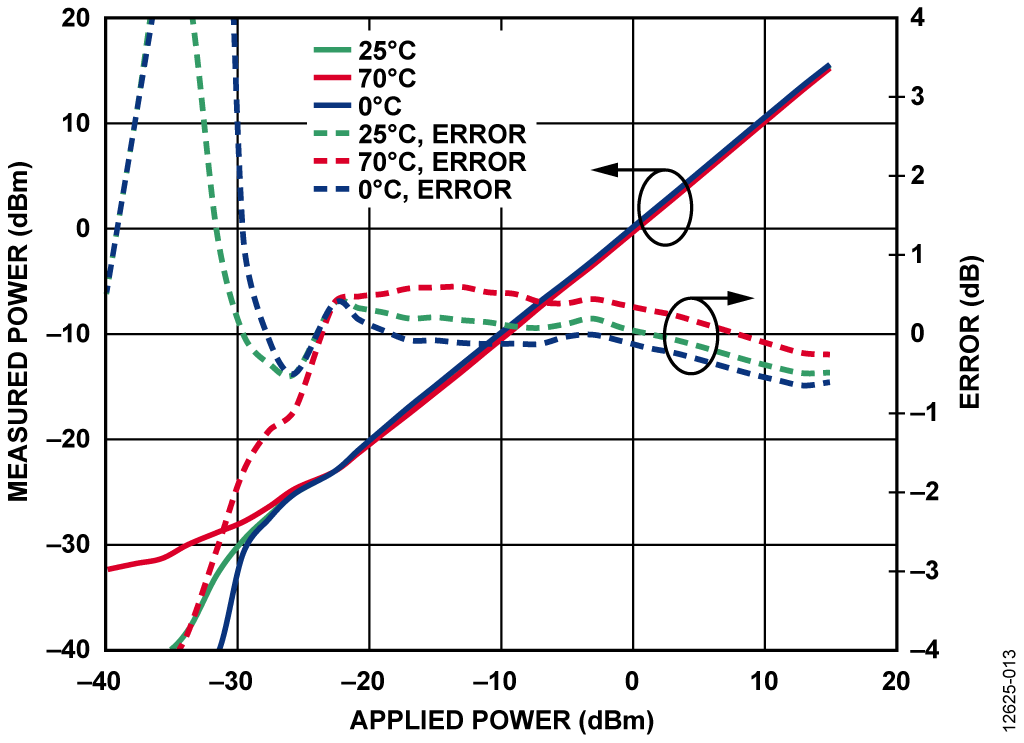Figure 13. Measured Power and Error vs. Applied Power for Various Temperatures at 30 GHz

### Summary of Equations

The following is a summary of the equations in the CN-0366, as well as additional equations to provide insight into the function of this power meter circuit.

The transfer function of the ADL6010 is given bywhere:

VOUT is the dc output voltage of the detector.
SlopeDET is the gain/slope of the detector, in V/V rms.
VIN is the rms voltage of the input RF signal.
INTDET is the intercept of the detector, in volts.

Solve for VIN usingThe ADC transfer function (when being driven by the ADL6010) is given by

CODE = VOUT/LSB
LSB = VREF/4096

where:

CODE is the ADC output code, a unitless number that can range from 0 and 4096.
VREF is the full-scale reference voltage of the ADC, in volts. LSB is the smallest quantized voltage that the ADC can resolve, in V/step or V/bit.

Solve for VOUT using

VOUT = CODE × LSB

Substituting this expression in the previous VIN equation yields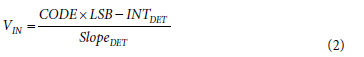Referring everything back to input power yields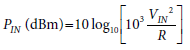where R is the impedance on the RFIN pin of the ADL6010. Substituting VIN into the power equation yieldsSimplifying the equation yields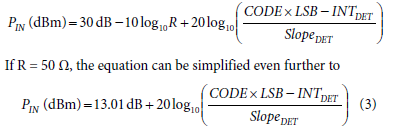This equation is in terms of the ADL6010 detector output slope and intercept, which is useful for conceptual purposes to show the interactions of the system. However, for practical purposes, the transfer function of the system is required in terms of the overall system slope and intercept, where the slope has units of codes/V and the intercept has units of codes. The final transfer function can be derived as follows.

The derivation of the system transfer function in terms of system slope (slopeSYS) and system intercept (INTSYS) begins with Equation 2, which has the input power in terms of detector slope (slopeDET) and detector intercept (INTDET). This derivation is achieved by multiplying both the numerator and denominator of the VIN expression (Equation 2) by 1/LSB, as follows: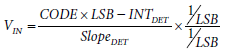Substituting this expression for VIN into Equation 2 yields Equation 1.

INTDET has units of volts, SlopeDET has units of V/V rms, and LSB has units of V/codes. Multiplying by 1/LSB converts INTDET into its equivalent ADC code and converts the detector slope into the system slope with unit of codes/V rms, yielding the following relations:

INTSYS = INTDET/LSB
Slopesys = SlopeDET/LSB

A complete set of design files, including schematics, layouts, Gerbers, and bill of materials for the CN-0366 circuit are available in the CN-0366 Design Support Package.

## Common Variations

In power monitoring and VGA applications, a common practice is to tap some power off of a transmission line with a coupler and then feed the signal into the RF/microwave detector, as shown in Figure 14.

When calibrating this setup, the previously described calibration routine does not change. The loss of the coupler and transmission lines are calibrated out and accounted for in the slope and intercept equations. Use the voltage level applied to the input of the coupler when computing the system slope and intercept. The system slope and intercept using the input voltage to the detector and the ADC output code are as follows: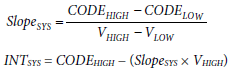where:

VHIGH, VLOW are the high and low voltages, respectively, applied to the input detector (generalized as VIN in Figure 14).
CODEHIGH, CODELOW are the high and low outputs of the ADC.

If a known voltage is applied to the input of the coupler (generalized as VCOUPLER in Figure 14), the general relationship between the input of the coupler and the input of the detector is

VIN = VCOUPLER × Voltage Loss

where Voltage Loss is a constant attenuation factor in fractional form.

For example, a 20 dB voltage loss from the input of the coupler to the input of the detector is a voltage loss factor of 1/10.

If the voltage levels at the input of the coupler are used instead of the input of the detector, a new expression for the system slope that includes the coupler and transmission line loss is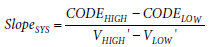where the VHIGH’ and VLOW’ are the high and low calibration voltages, respectively, at the input of the coupler.

The same equation in terms of the input voltages of the detector and the loss of the coupler and transmission lines is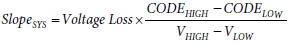Figure 15 shows the transfer function at 1 GHz and 5 GHz for a system using a coupler with 10 dB loss.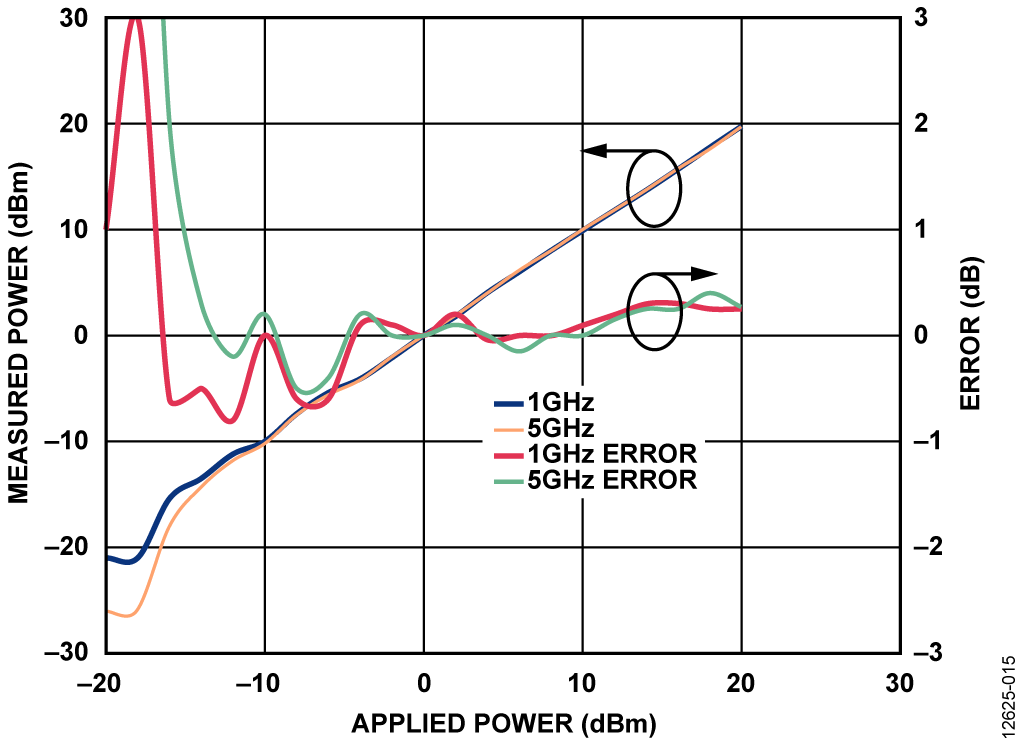Figure 15. Measured Power and Error vs. Applied Power at 1 GHz and 5 GHz for System Using 10 dB Coupler

## Circuit Evaluation & Test

### Equipment Needed

The following equipment is needed to perform the evaluations described in the CN-0366:

• The EVAL-SDP-CB1Z SDP board.
• The Agilent E8257D signal generator.
• The Agilent 34410A digital multimeter.
• A PC running Windows® 7 connected to the SDP board via a USB cable (supplied with the EVAL-SDP-CB1Z).
• A 5 V power supply to supply ADL6010-EVALZ board.
• A 9 V ac-to-dc, wall-mounted converter to supply the EVAL-AD7091RSDZ evaluation board (supplied with the EVAL-AD7091RSDZ). Note that the EVAL-SDP-CB1Z is powered from a regulator on the EVAL-AD7091RSDZ.
• The CN-0366 Evaluation Software.

### Getting Started

Make the following modifications and link settings to the ADL6010-EVALZ evaluation board and the EVAL-AD7091RSDZ evaluation board to implement the circuit shown in Figure 1.

For the ADL6010-EVALZ, replace R1 with a 200 Ω resistor (0402 size).

For the EVAL-AD7091RSDZ, replace R1 with a 0 Ω resistor (0603 size), and replace C13 with a 340 Ω resistor (0603 size). For the EVAL-AD7091RSDZ link settings, set LK1 to Position C, set LK2 to Position C, and leave LK3 and LK4 open.### Functional Block Diagram

Figure 16 shows the functional block diagram of the test setup that was used for testing the receive chain.

### Setup and Test

To set up and test the microwave power meter system, take the following steps:

1. Turn on all test equipment and wait for the test equipment to warm up.
2. Connect the ADL6010-EVALZ evaluation board input to the Agilent signal generator (connecting directly from the signal generator to the evaluation board via a barrel connector is recommended).
3. Connect the ADL6010-EVALZ evaluation board output to the input of the EVAL-AD7091RSDZ evaluation board.
4. Connect the EVAL-SDP-CB1Z SDP board to the EVAL-AD7091RSDZ evaluation board.
5. Connect the SDP board to a PC via the USB cable that is provided with the SDP board
6. Download and install the CN-0366 Evaluation Software onto the PC that is connected to the SDP control board.
7. After the software is installed properly, run the executable.
8. Turn on the signal generator and set it to a power and frequency within the operating limits of the ADL6010.
9. To obtain an accurate power reading, run the calibration routine in the software.
10. The software GUI now calculates and displays the correct power that is applied to the input of the ADL6010.

## Evaluation Boards

Pricing displayed is based on 1-piece.

Up to two boards can be purchased through Analog.com. To order more than two, please purchase through one of our listed distributors.

Pricing displayed is based on 1-piece. The USA list pricing shown is for budgetary use only, shown in United States dollars (FOB USA per unit), and is subject to change. International prices may vary due to local duties, taxes, fees and exchange rates.